``````int[] arr = new int;
``````

``````static void Main(string[] args)
{
int[] arr = new int;
for (int i = 0; i < 10; i++)
{
arr[i] = (int)Math.Pow(2, i);
}
// 显示数据
for (int i = 0; i < 10; i++)
{
Console.WriteLine(arr[i]);
}
}
``````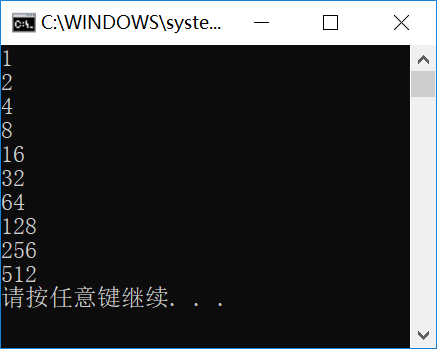``````static void Main(string[] args)
{
int[] arr = { 1, 1, 2, 3, 5 };
// 显示数据
foreach(int n in arr)
{
Console.WriteLine(n);
}
}
``````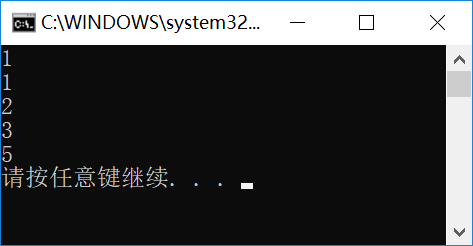## 多维数组

``````static void Main(string[] args)
{
int[,] matrix = new int[3,4];
//
for(int row=0;row<3;row++)
{
for(int col=0;col<4;col++)
{
Console.Write(" ({0},{1})", row, col);
}
Console.WriteLine();
}
}
``````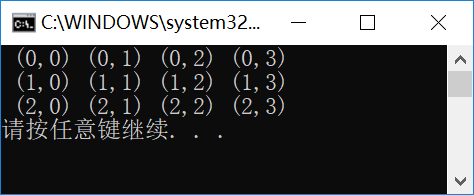``````static void Main(string[] args)
{
int[,] matrix = {
{ 1,2,3,4},
{5,6,7,8 },
{9,10,11,12 }
};
//
for (int row = 0; row < 3; row++)
{
for (int col = 0; col < 4; col++)
{
Console.Write(" ({0}) ", matrix[row, col]);
}
Console.WriteLine();
}
}
``````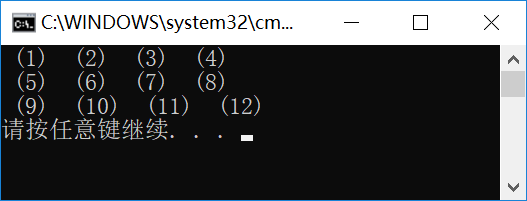## Array类

``````static void Main(string[] args)
{
int[,] matrix = {
{ 1,2,3,4},
{5,6,7,8 },
{9,10,11,12 }
};
Console.WriteLine("数组成员数量:{0}", matrix.Length);
Console.WriteLine("数组的维度:{0}", matrix.Rank);
Console.WriteLine("数组第一维的成员数量:{0}", matrix.GetLength(0));
}
``````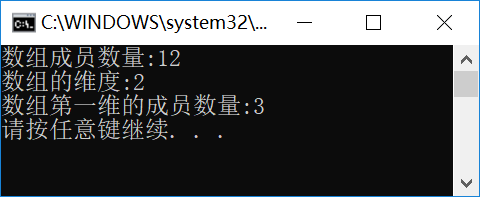• Length属性，返回数组成员的数量，请注意，这里是所有的成员，不考虑维度问题。此外，如果成员数量真的很多，还可以使用LongLength属性获取。
• Rank属性，返回数组的维度。
• GetLength()方法，返回指定维度的成员数量，请注意，第一维索引也是从0开始的。

## 自定义索引器

``````namespace ConsoleTest
{
public class CFibonacci
{
public int this[int n]
{
get
{
if (n == 1 || n == 2)
{
return 1;
}
else if (n > 2)
{
int x = 1, y = 1;
int tmp = 0;
for (int i = 3; i <= n; i++)
{
tmp = x;
x = y;
y += tmp;
}
return y;
}
else
{
return 0;
}
}
}
}
}
``````

``````static void Main(string[] args)
{
CFibonacci fib = new CFibonacci();
for(int i=1;i<=10;i++)
{
Console.WriteLine(fib[i]);
}
}
``````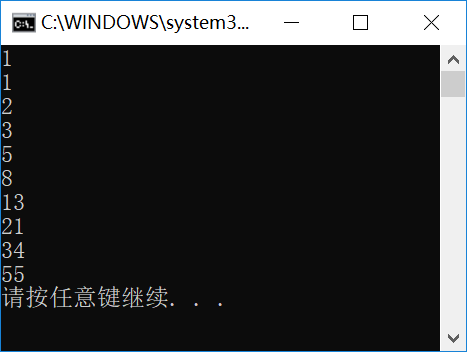``````public int[] GetSeq(int n)
{
if (n < 1) return new int;
//
int[] re = new int[n];
re = 1;
if (n > 1) re = 1;
//
if(n>2)
{
for(int i=2;i<n;i++)
{
re[i] = re[i - 2] +re[i - 1];
}
}
return re;
}
``````

``````static void Main(string[] args)
{
CFibonacci fib = new CFibonacci();
int[] arr = fib.GetSeq(10);
for (int i = 0; i < 10; i++)
{
Console.WriteLine(arr[i]);
}
}
``````CHY软件小屋原创作品！